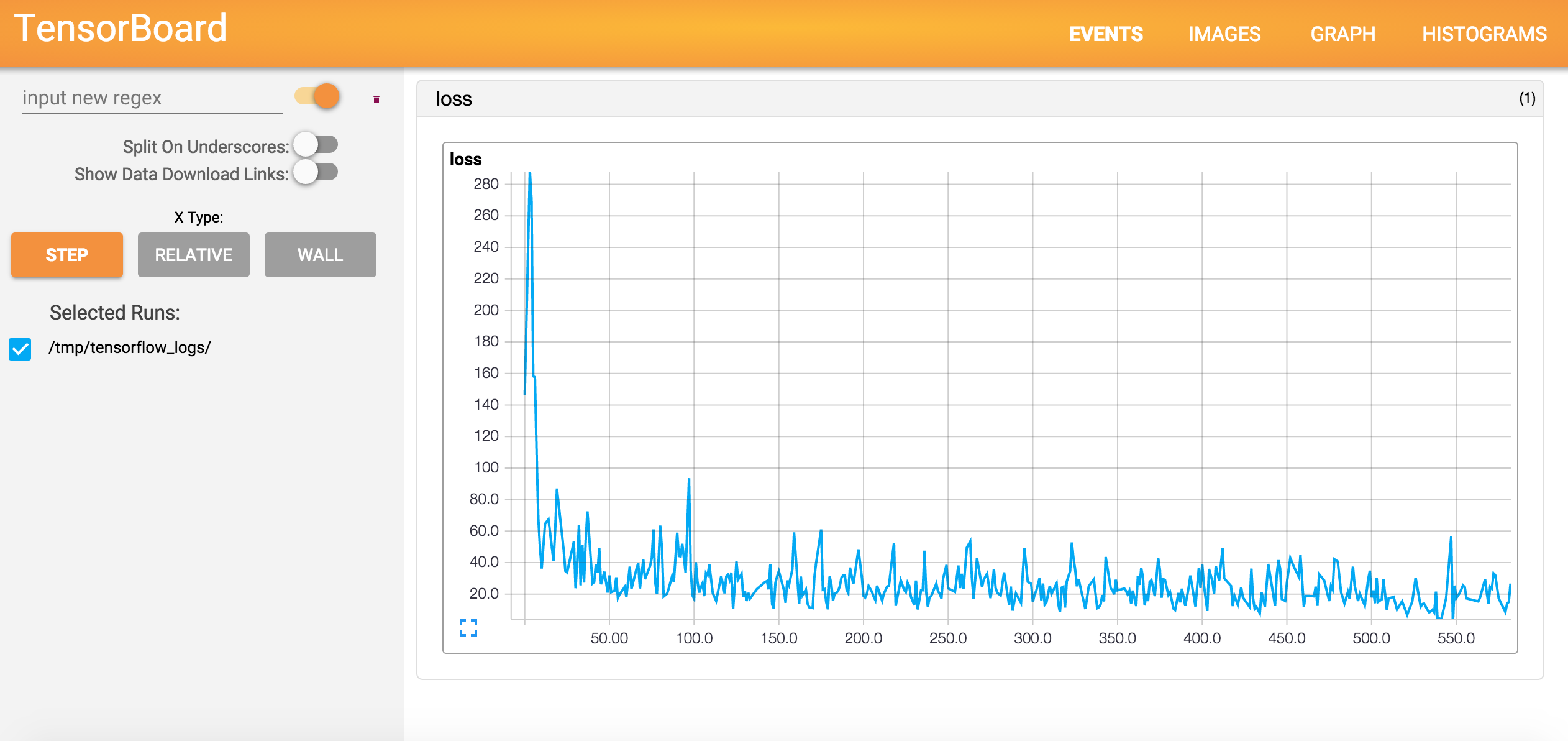# Loss Visualization in TensorFlow¶

Credits: Forked from TensorFlow-Examples by Aymeric Damien

## Setup¶

Refer to the setup instructions

In :
import tensorflow as tf
import numpy

# Import MINST data
import input_data

Extracting /tmp/data/train-images-idx3-ubyte.gz
Extracting /tmp/data/train-labels-idx1-ubyte.gz
Extracting /tmp/data/t10k-images-idx3-ubyte.gz
Extracting /tmp/data/t10k-labels-idx1-ubyte.gz

In :
# Use Logistic Regression from our previous example

# Parameters
learning_rate = 0.01
training_epochs = 10
batch_size = 100
display_step = 1

# tf Graph Input
x = tf.placeholder("float", [None, 784], name='x') # mnist data image of shape 28*28=784
y = tf.placeholder("float", [None, 10], name='y') # 0-9 digits recognition => 10 classes

# Create model

# Set model weights
W = tf.Variable(tf.zeros([784, 10]), name="weights")
b = tf.Variable(tf.zeros(), name="bias")

# Construct model
activation = tf.nn.softmax(tf.matmul(x, W) + b) # Softmax

# Minimize error using cross entropy
cost = -tf.reduce_sum(y*tf.log(activation)) # Cross entropy

# Initializing the variables
init = tf.initialize_all_variables()

In :
# Create a summary to monitor cost function
tf.scalar_summary("loss", cost)

# Merge all summaries to a single operator
merged_summary_op = tf.merge_all_summaries()

In [ ]:
# Launch the graph
with tf.Session() as sess:
sess.run(init)

# Set logs writer into folder /tmp/tensorflow_logs
summary_writer = tf.train.SummaryWriter('/tmp/tensorflow_logs', graph_def=sess.graph_def)

# Training cycle
for epoch in range(training_epochs):
avg_cost = 0.
total_batch = int(mnist.train.num_examples/batch_size)
# Loop over all batches
for i in range(total_batch):
batch_xs, batch_ys = mnist.train.next_batch(batch_size)
# Fit training using batch data
sess.run(optimizer, feed_dict={x: batch_xs, y: batch_ys})
# Compute average loss
avg_cost += sess.run(cost, feed_dict={x: batch_xs, y: batch_ys})/total_batch
# Write logs at every iteration
summary_str = sess.run(merged_summary_op, feed_dict={x: batch_xs, y: batch_ys})
# Display logs per epoch step
if epoch % display_step == 0:
print "Epoch:", '%04d' % (epoch+1), "cost=", "{:.9f}".format(avg_cost)

print "Optimization Finished!"

# Test model
correct_prediction = tf.equal(tf.argmax(activation, 1), tf.argmax(y, 1))
# Calculate accuracy
accuracy = tf.reduce_mean(tf.cast(correct_prediction, "float"))
print "Accuracy:", accuracy.eval({x: mnist.test.images, y: mnist.test.labels})


### Run the command line¶

tensorboard --logdir=/tmp/tensorflow_logs

# Loss per minibatch step